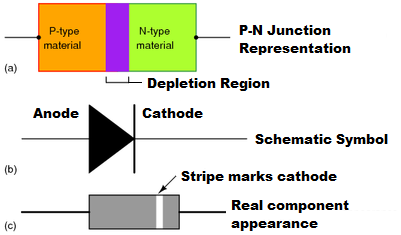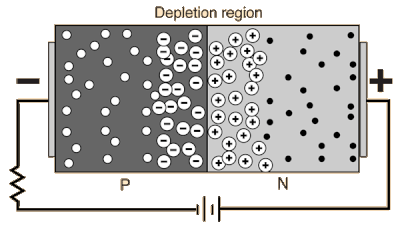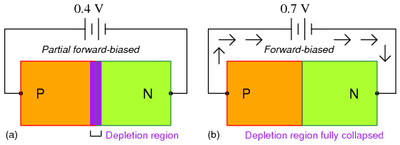# Diode operationFigure 1. The p-n junction of a diode along with its corresponding schematic and real component. The cathode and the anode of the diode are labelled so that conventional current flows from anode to cathode through the diode.

The way that a diode operates can be difficult to understand as it involves fairly advanced quantum mechanics. However, at the simplest level the operation of a diode can be understood by looking at the flow of positive charges (or "holes") and the negative charges (the electrons). Technically, a semiconductor diode is referred to as a p-n junction. These p-n junctions are important in the operation of a photovoltaic cell as well. Having the diode work properly requires a process known as doping. Semiconductors can be doped with materials so that they have an excess of easily displaced electrons - generally referred to as a negative or n-type region. As well, they can be doped with elements that create an excess of holes that easily absorb these electrons - generally referred to as a positive or p-type region. The negative and positive regions of the diode are also the component's cathode and anode respectively (see Figure 1).

The differences between these two materials and their interactions over very short distances (less than a millimeter) result in a diode when the two types are joined. Joining these two types creates the p-n junction, and the region between the two sides is called the depletion region, as electrons from the n-type region diffuse and fill some of the holes in the p-type region. This creates negative ions in the p-type region and leaves behind positive ions in the n-type region (see Figure 2). This responds to electric fields differently depending on the direction of the electric field. This leads to useful electronic behaviour depending on which way the voltage (or electric field) is applied, this is called biasing.

## Bias

A diode (PN junction) in an electrical circuit allows current to flow more easily in one direction than another. Forward biasing means putting a voltage across a diode that allows current to flow easily, while reverse biasing means putting a voltage across a diode in the opposite direction. The voltage with reverse biasing doesn't cause any appreciable current to flow. This is useful for changing AC current to DC current. It has other uses in manipulating electronic signals as well.

### Reverse BiasFigure 2. A reverse-biased p-n junction with the black circles representing easily displaced electrons and the white circles representing electron-deficient "holes." In a reverse-biased junction such as this, the electrons would leave the black circles and move toward the external circuit, leaving behind more positive ions, while electrons from the external circuit would "fill the holes," creating more negative ions.

If a voltage is applied across the diode in such a way that the n-type half of the diode was connected to the positive terminal of the voltage source and the p-type half was connected to the negative terminal, electrons from the external circuit would create more negative ions in the p-type region by "filling the holes" and more positive ions would be created in the n-type region as electrons are displaced toward the positive terminal of the voltage source (see Figure 2). Hence, the depletion region would increase and the voltage between the p-type and n-type regions would also increase as the total charge on each side of the junction increases in magnitude until the voltage across the diode equals and opposes the applied voltage and cancels it out, ceasing the current through the circuit. This process happens nearly instantaneously and results in essentially no current flow through the circuit when voltage is applied in this direction across the diode. This is known as a reverse-biased p-n junction.

### Forward BiasFigure 3. A partially and fully forward-biased p-n junction. Note that a minimum voltage is required to collapse the depleted region.

When the voltage is applied in the opposite direction across the diode, the depletion region begins to shrink (see Figure 3). In a reverse-biased diode, the electrons and holes would be pulled away from the junction, but a forward-biased scenario ensures that the electrons and holes move toward the junction as they are repelled from the positive and negative terminals of the voltage source respectively. Given a great enough applied voltage, both the holes and the electrons would overcome the depletion region and meet near the junction, where they could combine in a continuous process, closing the circuit and allowing current flow.

### Forward Voltage and Breakdown Voltage

There is a minimum threshold voltage required to overcome the depletion region, which for most silicon diodes is a significant 0.7 volts. Furthermore, reverse-bias voltage does induce a small amount of current through the diode called leakage current that is essentially negligible for most purposes. Finally, a great enough reverse voltage will result in the complete electronic breakdown of the diode and allow current to flow through the diode in the reverse direction.